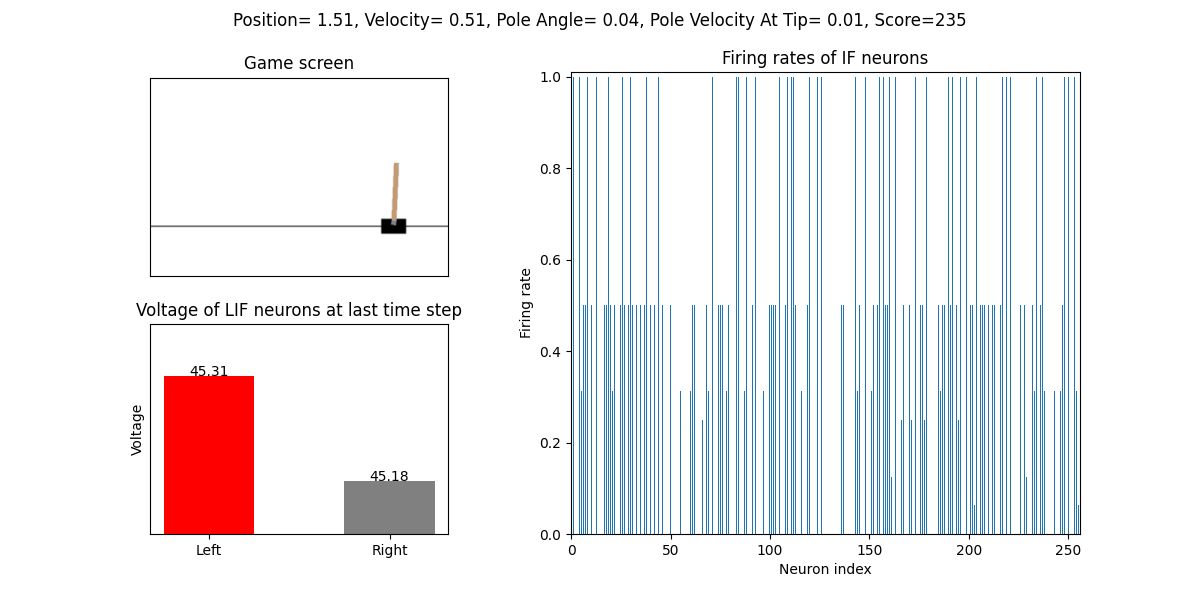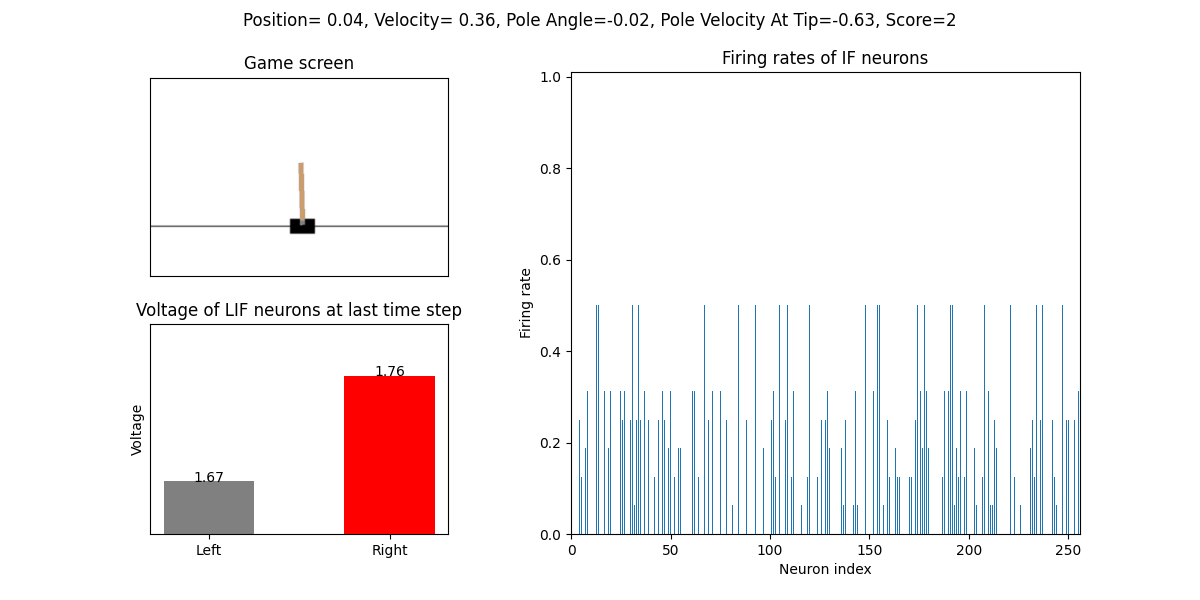# Reinforcement Learning: Deep Q Learning

Authors: fangwei123456lucifer2859

Translator: LiutaoYu

This tutorial applies a spiking neural network to reproduce the PyTorch official tutorial REINFORCEMENT LEARNING (DQN) TUTORIAL. Please make sure that you have read the original tutorial and corresponding codes before proceeding.

## Change the input

In the ANN version, the difference between two adjacent frames of CartPole is directly used as input, and then CNN is used to extract features. We can also use the same method for the SNN version. However, to obtain the frames, the graphical interface must be activated, which is not convenient for training on a remote server without a graphical interface. To reduce the difficulty, we directly use CartPole’s state variables as the network input, which is an array containing 4 floating numbers, i.e., Cart Position, Cart Velocity, Pole Angle and Pole Velocity At Tip. The training code also needs to be changed accordingly, which will be shown below.

Next, we need to define the SNN structure. Usually in Deep Q Learning, the neural network acts as the Q function, the output of which should be continuous values. This means that the last layer of the SNN should not output spikes representing Q function as 0 and 1, which may lead to poor performance. There are several methods to making SNN output continuous values. For the classification tasks in the previous tutorials, the final output of the network is the firing rate of each neuron in the output layer, which is obtained by counting the number of spikes in the simulation duration and then dividing the number by the duration. Through preliminary testing, we found that using firing rate as Q function can not lead to satisfying performance. Because after simulating $$T$$ steps, the possible firing rates are $$0, \frac{1}{T}, \frac{2}{T}, ..., 1$$, which are not enough to represent the Q function.

Here, we apply a new method to make SNN output floating numbers. We set the firing threshold of a neuron to be infinity, which won’t fire at all, and we adopt the final membrane potential to represent Q function. It is convenient to implement such neurons in the SpikingJelly framework: just inherit everything from LIF neuron neuron.LIFNode and rewrite its forward function.

class NonSpikingLIFNode(neuron.LIFNode):
def forward(self, dv: torch.Tensor):
self.neuronal_charge(dv)
# self.neuronal_fire()
# self.neuronal_reset()
return self.v


The structure of the Deep Q Spiking Network is very simple: input layer, IF neuron layer, and NonSpikingLIF neuron layer, between which are fully linear connections. The IF neuron layer is an encoder to convert the CartPole’s state variables to spikes, and the NonSpikingLIF neuron layer can be regraded as the decision making unit.

class DQSN(nn.Module):
def __init__(self, input_size, hidden_size, output_size, T=16):
super().__init__()
self.fc = nn.Sequential(
nn.Linear(input_size, hidden_size),
neuron.IFNode(),
nn.Linear(hidden_size, output_size),
NonSpikingLIFNode(tau=2.0)
)

self.T = T

def forward(self, x):
for t in range(self.T):
self.fc(x)

return self.fc[-1].v


## Training the network

The code of this part is almost the same with the ANN version. But note that the SNN version here adopts Observation returned by env as the input.

Following is the training code of the ANN version:

for i_episode in range(num_episodes):
# Initialize the environment and state
env.reset()
last_screen = get_screen()
current_screen = get_screen()
state = current_screen - last_screen
for t in count():
# Select and perform an action
action = select_action(state)
_, reward, done, _ = env.step(action.item())
reward = torch.tensor([reward], device=device)

# Observe new state
last_screen = current_screen
current_screen = get_screen()
if not done:
next_state = current_screen - last_screen
else:
next_state = None

# Store the transition in memory
memory.push(state, action, next_state, reward)

# Move to the next state
state = next_state

# Perform one step of the optimization (on the target network)
optimize_model()
if done:
episode_durations.append(t + 1)
plot_durations()
break
# Update the target network, copying all weights and biases in DQN
if i_episode % TARGET_UPDATE == 0:


Here is training code of the SNN version. During the training process, we will save the model parameters responsible for the largest reward.

for i_episode in range(num_episodes):
# Initialize the environment and state
env.reset()
state = torch.zeros([1, n_states], dtype=torch.float, device=device)

total_reward = 0

for t in count():
action = select_action(state, steps_done)
steps_done += 1
next_state, reward, done, _ = env.step(action.item())
total_reward += reward
next_state = torch.from_numpy(next_state).float().to(device).unsqueeze(0)
reward = torch.tensor([reward], device=device)

if done:
next_state = None

memory.push(state, action, next_state, reward)

state = next_state
if done and total_reward > max_reward:
max_reward = total_reward
torch.save(policy_net.state_dict(), max_pt_path)
print(f'max_reward={max_reward}, save models')

optimize_model()

if done:
print(f'Episode: {i_episode}, Reward: {total_reward}')
writer.add_scalar('Spiking-DQN-state-' + env_name + '/Reward', total_reward, i_episode)
break

if i_episode % TARGET_UPDATE == 0:


It should be emphasized here that, we need to reset the network after each forward process, because SNN is retentive while each trial should be started with a clean network state.

def select_action(state, steps_done):
...
if sample > eps_threshold:
ac = policy_net(state).max(1).view(1, 1)
functional.reset_net(policy_net)
...

def optimize_model():
...
state_action_values = policy_net(state_batch).gather(1, action_batch)

next_state_values = torch.zeros(BATCH_SIZE, device=device)
functional.reset_net(target_net)
...
optimizer.step()
functional.reset_net(policy_net)


The integrated script can be found here clock_driven/examples/Spiking_DQN_state.py. And we can start the training process in a Python Console as follows.

>>> from spikingjelly.clock_driven.examples import Spiking_DQN_state
>>> Spiking_DQN_state.train(use_cuda=False, model_dir='./model/CartPole-v0', log_dir='./log', env_name='CartPole-v0', hidden_size=256, num_episodes=500, seed=1)
...
Episode: 509, Reward: 715
Episode: 510, Reward: 3051
Episode: 511, Reward: 571
complete
state_dict path is./ policy_net_256.pt


## Testing the network

After training for 512 episodes, we download the model policy_net_256_max.pt that maximizes the reward during the training process from the server, and run the play function on a local machine with a graphical interface to test its performance.

>>> from spikingjelly.clock_driven.examples import Spiking_DQN_state
>>> Spiking_DQN_state.play(use_cuda=False, pt_path='./model/CartPole-v0/policy_net_256_max.pt', env_name='CartPole-v0', hidden_size=256, played_frames=300)


The trained SNN controls the left or right movement of the CartPole, until the end of the game or the number of continuous frames exceeds played_frames. During the simulation, the play function will draw the firing rate of the IF neuron, and the voltages of the NonSpikingLIF neurons in the output layer at the last moment, which directly determine the movement of the CartPole.The performance after 16 episodes:The performance after 32 episodes:The reward increases with training:Here is the performance of the ANN version (The code can be found here clock_driven/examples/DQN_state.py).